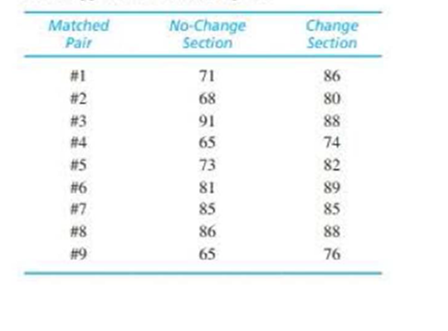Chapter 11, Problem 21PEssentials of Statistics for the B...

8th Edition
Frederick J Gravetter + 1 other
ISBN: 9781133956570

Solutions

Chapter
SectionEssentials of Statistics for the B...

8th Edition
Frederick J Gravetter + 1 other
ISBN: 9781133956570
Textbook Problem

Some evidence suggests that you are likely to improve your test score if you rethink and change answers on a multiple-choice exam (Johnston. 1975). To examine this phenomenon, a teacher gave the same final exam to two sections of a course. Students in one section were told to turn in their exams immediately after finishing, without changing any answers. In the other section, students were encouraged to reconsider each question and to change answers when they felt it was appropriate. Before the final, the teacher matched 9 students in the first section with 9 students in the second section based on their midterm grades. For example, a student in the no-change section with an 89 cm the midterm was matched with a student in the change section who also had an 89 on the midterm. The final exam grades for the 9 matched pairs of students are presented in the table below.a. Do the data indicate a significant difference between the two conditions? Use a two-tailed test with α = .05.b. Construct a 95% confidence interval to estimate the size of the population mean difference.c. Write a sentence demonstrating how the results of the hypothesis test and the confidence interval would appear in a research report.a.

To determine
Whether there is a significant difference between the scores for the section with change and the section with no change.

Explanation

Given info:

The given data are shown below,

 Matched pair No-Change Section Change Section #1 71 86 #2 68 80 #3 91 88 #4 65 74 #5 73 82 #6 81 89 #7 85 85 #8 86 88 #9 65 76

Calculation:

The difference D, D and D2 are obtained below:

 Matched pair No-Change Section Change Section D D2 #1 71 86 -15 225 #2 68 80 -12 144 #3 91 88 3 89 #4 65 74 -9 81 #5 73 82 -9 81 #6 81 89 -8 64 #7 85 85 0 0 #8 86 88 -2 4 #9 65 76 -11 121 Total ∑D=63 ∑D2=729

Thus, the values of D is 63 and D2 is 729.

The total number of pairs considered is n=9.

The mean difference MD:

MD=DN=639=7

Thus, the mean difference MD is 7.

The value of sum of squares,SS is,

SS=D2(D)2n=7296329=729441=288

Thus, the value of SS is 30

b.

To determine
The confidence interval to estimate the score difference at 95 %.

c.

To determine

To write: A sentence demonstrating the way the results of the hypothesis test and confidence interval would appear in a research report.

Still sussing out bartleby?

Check out a sample textbook solution.

See a sample solution

The Solution to Your Study Problems

Bartleby provides explanations to thousands of textbook problems written by our experts, many with advanced degrees!

Get Started

In Exercises 1728, use the logarithm identities to obtain the missing quantity.

Finite Mathematics and Applied Calculus (MindTap Course List)

The vector represented by where A(4, 8) and B(6, 6)is:

Study Guide for Stewart's Multivariable Calculus, 8th

True or False: is a convergent series.

Study Guide for Stewart's Single Variable Calculus: Early Transcendentals, 8th